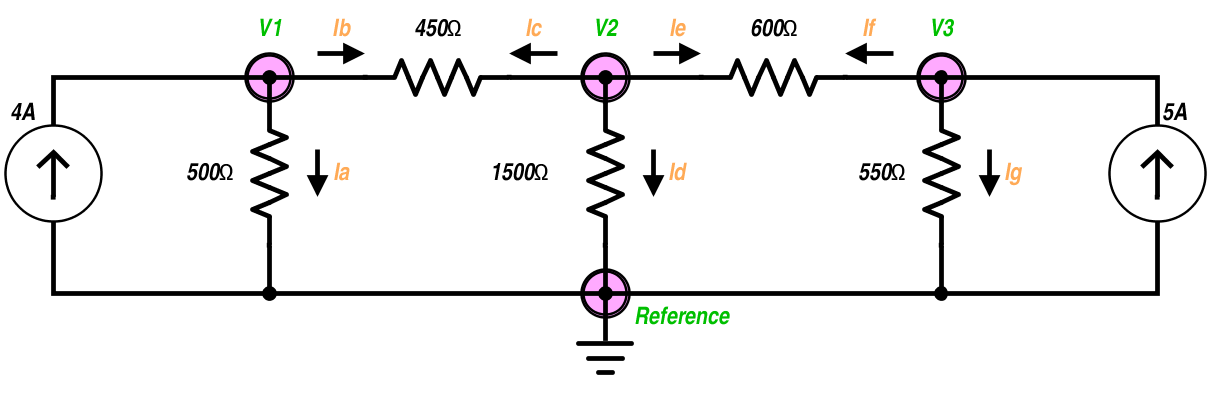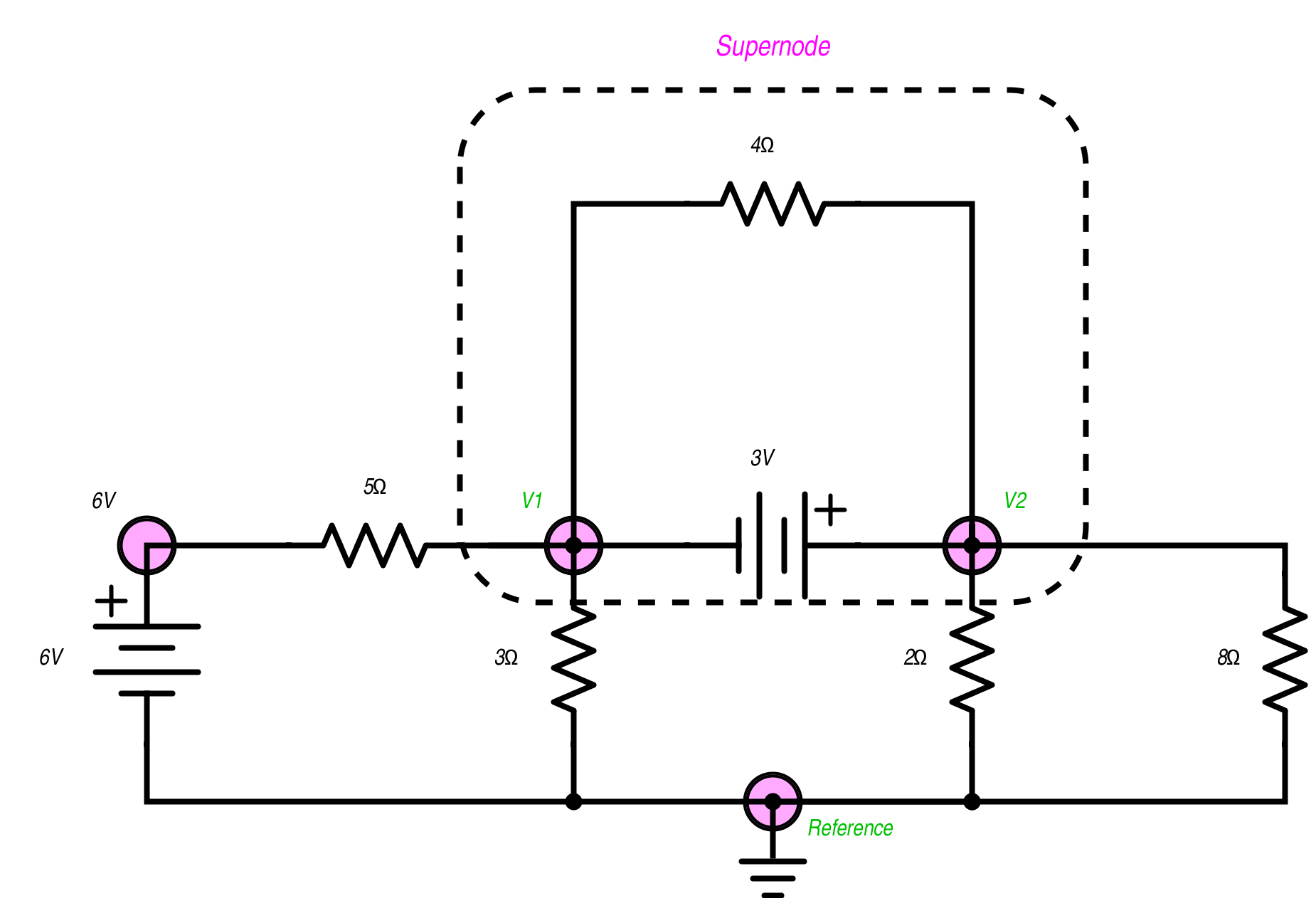Technical Article

# Special Cases in Nodal Analysis

July 09, 2015 by Tristan Cornell

#### Recommended Level

Beginner

When we are designing electronic circuits, it is always important to know how much current is flowing through a component or how much voltage is present at a particular node in the circuit at crucial points in its operation. Finding either measurement can be done using Kirchhoff’s circuit laws. The two analysis types that allow us to find these values are Mesh Analysis and Nodal Analysis. If we are seeking to find the voltage at a point (node), then we can apply nodal analysis using Kirchhoff’s Current Law (KCL).Each specific node in this schematic (V1, V2, and V3) has 3 connections. KCL declares that the sum of all branch currents from each node is zero. We can use this to find the voltage at each node by the following method:

First, we have a reference node with the lowest potential which will be called the ground. The ground in this circuit is chosen because it is a common point with the lowest voltage. Next, we assign a variable to each node where the voltage is unknown. This is marked by the circles at V1, V2, and V3.Third, apply KCL to form an equation for each unknown voltage.

For node V1:

The currents Ia and Ib:

$$Ia$$ = $$\frac{V1}{500Ω}$$ and $$Ib =\frac{(V1-V2)}{450Ω}$$

It is because the voltage thru the resistor is the difference of potential between its two nodes. Since V1 is the only node directly connected to the 4 amp current source, $$Ia + Ib = 4 Amps$$.

Putting this all together:

$$\frac{V1}{500Ω} + \frac{(V1-V2)}{450Ω} = 4 Amps$$.

This can be rewritten as:

$$V1(\frac{1}{500Ω}+\frac{1}{450Ω}) - V2(\frac{1}{450Ω})=4 Amps$$.

For node V2:

Ic is pointing from V2 to V1 so we will write the 450Ω resistor branch as: $$\frac{(V2-V1)}{450Ω}$$.

Id is simply: $$\frac{V2}{1500Ω}$$.

Ie flows from V2 to V3 and it is noted as: $$\frac{(V2-V3)}{600Ω}$$.

Remember that KCL requires the sum of all 3 branches to be zero. This means $$Ic+Id+Ie=0$$.

As one formula, it is put together as:

$$\frac{(V2-V1)}{450Ω} + \frac{V2}{1500Ω} + \frac{(V2-V3)}{600Ω} = 0$$.

A friendlier form for linear equations would be:

$$-V1(\frac{1}{450})+V2(\frac{1}{450}+\frac{1}{1500}+\frac{1}{600})-V3(\frac{1}{600})=0$$.

Node V3 is the same construction as node V1, only with different values.

Ig is: $$\frac{V3}{550 Ω}$$.

If (eye-eff, not iff. English mocks us!) is: $$\frac{(V3-V2)}{600 Ω}$$.

Both resistors are fed from the 5-Amp source, making $$If+Ig=5 A$$.

Put together, we have:

$$\frac{(V3-V2)}{600 Ω} + \frac{V3}{550 Ω}=5 A$$.

Prettied up for calculation, the equation is:

$$–V2(\frac{1}{600})+V3(\frac{1}{550}+\frac{1}{600})=5$$.

The fourth and last step is to solve the system of equations. There are calculators that can solve systems of linear equations. Matlab and GNU Octave are pc programs that can perform this function. With a pencil, paper, and 20 minutes of time; we could solve this “Old School” using Algebra. However we might as well use a faster and possibly more reliable method, so let’s go with an online option of www.wolframalpha.com.

Our three final equations can be grouped together as:

$$v1(\frac{1}{500}+\frac{1}{450} - v2(\frac{1}{450})=4$$,

$$-v1(\frac{1}{450}) + v2(\frac{1}{450}+\frac{1}{1500}+\frac{1}{600})-v3(\frac{1}{600})=0$$,

$$–v2(\frac{1}{600})+v3(\frac{1}{550}+\frac{1}{600})=5$$.

Though this is mathematically correct, WolframAlpha basically replied with “huh”?.

To make the formula a little more agreeable, let’s throw in “*” for multiplication:

$$v1 * (\frac{1}{500}+\frac{1}{450} - v2 * (\frac{1}{450})= 4$$,

$$-v1 * (\frac{1}{450}) + v2 * (\frac{1}{450}+\frac{1}{1500}+\frac{1}{600}) - v3 * (\frac{1}{600})=0$$,

$$–v2 * (\frac{1}{600}) + v3 * (\frac{1}{550}+\frac{1}{600})=5$$.

The solution is a bit messy as

$$\underline{v1= \frac{3159000}{1697}}$$.

But clicking approximate form on the web page will yield:

$$\underline{v1=1,861.5}$$, $$\underline{v2=1,736.9}$$ and $$\underline{v3=2,265.5}$$.

To check this, compare power flowing into the circuit from both sources to power being dissipated by the resistors. The node V1 has 1,861.5 Volts with 4 Amps equaling 7,446 Watts. At 2,265.5 Volts @ 5 Amps, node V3 has 11,327.5 Watts. Resistors are producing heat at the following rate: 450 Ω 34.5 Watts, 500 Ω 6,930.36 Watts, 1500 Ω 2,011.21 Watts, 600 Ω 465.7 Watts, and 550 Ω 9,331.8 Watts. Power in is 18,773.5 Watts. Power dissipated is 18,773.57 Watts due to rounding issues. Either we have designed the world’s most powerful toaster oven, or our current should been a bit less for this example!

Special Cases: Voltage Sources and Supernodes.The addition of voltage sources presents a special case situation. Here we have a 6 volt source and 3 volt source. The 3 volt source is between two non-reference nodes and forms a supernode.

Finding the reference node is same process as it was in the last example.

Now things change a little bit. The 6V node does not require KCL because we already know the circuit is 6 volts at this location. The supernode is not as bad as it looks, we just need to have a KVL equation added. The V2 side of the 3 volt battery has a higher voltage potential than the V1 side, so the KVL we will use is $$V2 - V1 = 3V$$.

The KCL for the rest of the circuit is:

$$\frac{(V1-6v)}{5 Ω} + \frac{V1}{3 Ω} + \frac{V2}{2 Ω} + \frac{V2}{8 Ω} = 0$$.

You may have noticed that the math isn’t as messy in this example. We chose to divide by the resistance rather than multiply by the reciprocal. Either way is perfectly valid.

Hey! What about the 4 Ω resistor? No one wants to be left out! Well, the 4 Ω resistor is part of a package deal. It is seen as part of the supernode and does not have to be factored in as a separate equation. Lucky us!

We can add a few parentheses to our linear equations to make things a bit more clear and input them into the WolframAlpha page as:

$$v2-v1=3$$, $$\frac{(v1-6)}{5}+\frac{(v1)}{3}+\frac{(v2)}{2}+\frac{(v2)}{8}=0$$.

Lo and behold, we find: $$\underline{V1 = -0.5827}$$ and $$\underline{V2 = 2.4173}$$ as our answer.

As complex as this may seem, nodal analysis is the basis for many circuit simulation programs and is a cornerstone for understanding voltages at intersecting points in a circuit.

1 Comment• T
think twice February 26, 2016

Hello Tristan,
concerning the first circuit with current source try to solve it with other method to find the appropriate answer,
like solving the same circuit with superposition method will lead to the same results in voltage and current as nodal analysis ,
so this circuit is not a special case.

Like.